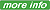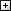Processing ......FreeComputerBooks.com Free Computer, Mathematics, Technical Books and Lecture Notes, etc.

Calculus and Mathematical Analysis
Flight Visualizer, track Un-filtered flights all over the world!
:Mathematics and Logic BooksAlgebra and Linear Algebra BooksProbability and Stochastic BooksStatistics BooksNumerical Computation BooksGraph Theory BooksAn Introduction to Measure Theory (Terrence Tao)Analysis and Linear Algebra for Finance (Patrick Roger)Precalculus: An Investigation of Functions ©2011 (David Lippman and Melonie Rasmussen)Elementary Calculus: An Infinitesimal Approach ©1985,2000,2010 (H. Jerome Keisler) 992 pagesMathematical Analysis II ©2009 (Elias Zakon) 424 pagesIntroduction to Real Analysis ©2002-2009 (William F. Trench) 574 pagesUnderstanding Calculus ©2009 (Faraz Hussain)Vector Calculus ©2009 (Michael Corral) 222 pagesCalculus, Applications and Theory ©2008 (Kenneth Kuttler) 912 pages [Homepage]Calculus I ©2007 (Paul Dawkins)Calculus II ©2007 (Paul Dawkins)Calculus III ©2007 (Paul Dawkins)The Calculus of Functions of Several Variables ©1999-2001 (Dan Sloughter)Vector Calculus ©2008 (Michael Corral) 222 pagesComplex Variables - Complex AnalysisYet Another Calculus Text - an Short Introduction with Infinitesimals ©2007 (Dan Sloughter) 187 pages [Mirror]Mathematical Background: Foundations of Infinitesimal Calculus ©2003 (Keith Duncan Stroyan) 182 pagesCalculus Without Limits ©1999 (Karl Heinz Dovermann) 302 pagesAdvanced Calculus ©1990 (Lynn H. Loomis/Shlomo Sternberg, Harvard University) 592 pagesElementary Calculus: An Infinitesimal Approach ©1985 (H. Jerome Keisler) 913 pages [PDF]Multivariable Calculus ©1996-1997 (George Cain and James Herod)Multivariable Calculus, Applications and Theory ©2006 (Kenneth Kuttler) 474 pages [Homepage]Free Calculus Notes (Ethan Duckworth)The Calculus Bible, 370 pages [Homepage]Mathematical Analysis I - Real Analysis for Undergraduates (Elias Zakon)Complex Analysis (George Cain)Complex Variables (Robert B. Ash/W.P. Novinger)
Book CategoriesAll CategoriesRecent BooksIT Research LibraryMiscellaneous BooksComputer LanguagesComputer ScienceData Science/DatabasesElectronic EngineeringJava and Java EE (J2EE)Linux and UnixMathematicsMicrosoft and .NETMobile ComputingNetworking and CommunicationsSoftware EngineeringSpecial TopicsWeb Programming
Other Categories
Resources and Links